• SVD奇异值分解
• SVD在推荐算法中的应用
• PCD 数据降维
• 一个图片处理的例子

SVD奇异值分解

SVD奇异值分解和特征分解很像，区别就是此时Data 是一个m * n的矩阵，不是方阵。ΣVT就分别是m* m、m*n和n*n。Σ为对角矩阵，对角元素称为奇异值。

SVD特征分解的关系：

Data * DataT 是个方阵，特征分解可得  Data * DataT  = U mxm *Σ1* UT mxm

DataT * Data  是个方阵，特征分解可得 DataT * Data = V nxn *Σ2* VTnxn

svd 分解中 U mxm 就是Data * DataT 的特征向量，V nxn 就是DataT * Data 的特征向量Σ Data * DataT 特征值Σ1的平方根。

SVD的几个特性

１：取特征值的前Ｋ个项，可以近似的表示矩阵Data。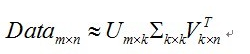２：取特征值的前ｋ项，Ｖｎｘｋ可以表示为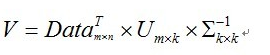SVD在推荐算法中的应用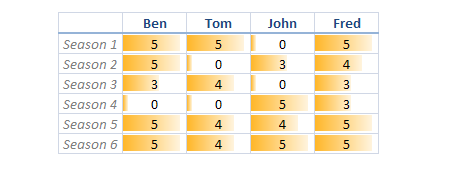U =
-0.4472   -0.5373   -0.0064   -0.5037   -0.3857   -0.3298
-0.3586    0.2461    0.8622   -0.1458    0.0780    0.2002
-0.2925   -0.4033   -0.2275   -0.1038    0.4360    0.7065
-0.2078    0.6700   -0.3951   -0.5888    0.0260    0.0667
-0.5099    0.0597   -0.1097    0.2869    0.5946   -0.5371
-0.5316    0.1887   -0.1914    0.5341   -0.5485    0.2429

S =
17.7139         0         0         0
0    6.3917         0         0
0         0    3.0980         0
0         0         0    1.3290
0         0         0         0
0         0         0         0

V =
-0.5710   -0.2228    0.6749    0.4109
-0.4275   -0.5172   -0.6929    0.2637
-0.3846    0.8246   -0.2532    0.3286
-0.5859    0.0532    0.0140   -0.8085

矩阵 U 的每一行，反映的是每一个商品的特征。矩阵 V 的每一行，反映的是每一个用户的特征。

保留2个维度的的特征，再对三个矩阵相乘。可以得到原始近似值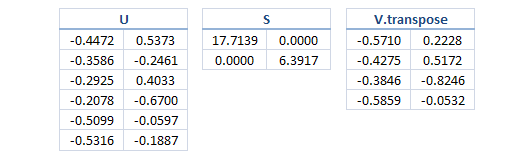U(1:6,1:2) * S(1:2,1:2) * V(1:4,1:2)' 得到的新矩阵和原始矩阵相似。

这个就是前面提到的svd特性1，但这个不是我们在推荐系统中要用到的特性，接下来的图片处理的例子将用到这个特性。

5.2885    5.1627    0.2149    4.4591
3.2768    1.9021    3.7400    3.8058
3.5324    3.5479   -0.1332    2.8984
1.1475   -0.6417    4.9472    2.3846
5.0727    3.6640    3.7887    5.3130
5.1086    3.4019    4.6166    5.5822

为了更形象的描述 特征 矩阵能反映出商品和用户 的特征。把前面保留2个维度的 U，V 矩阵列 表示在 一张二维图上。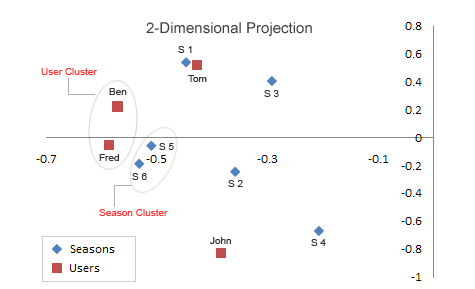s5,s6 也在差不多的位置上，对比原始矩阵它们的评分也基本相近。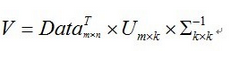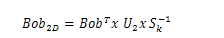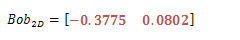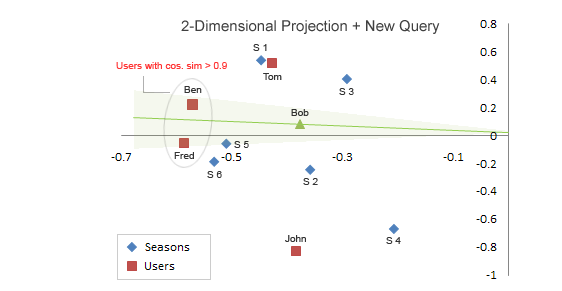svd 在里面的关键作用是：

1：自动帮我们划分出基于用户 的特征，和基于商品的特征。

2：在上面的基础上，选取一个维度K。使用我们可以在一个低维空间下计算相似度，降低运算复杂度。

PCA 数据降维

Principal Component Analysis 主成份分析，这个是机器学习常用的数据降维方法。

pca 的作用就是保留主要的特征维度，去掉不必要的特征，更加有效的提高和优化我们的学习算法。

pca算法原理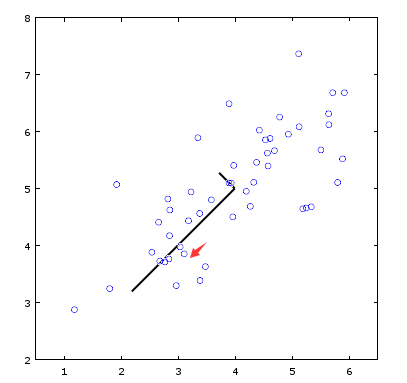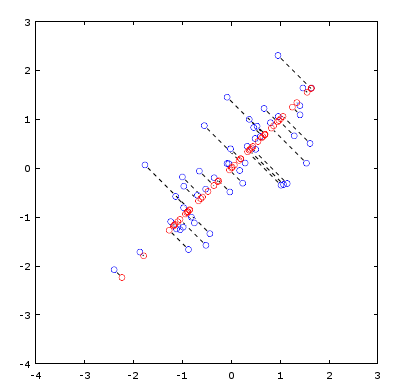PCA 算法步骤，octave 代码

displayData(X(1:100, :));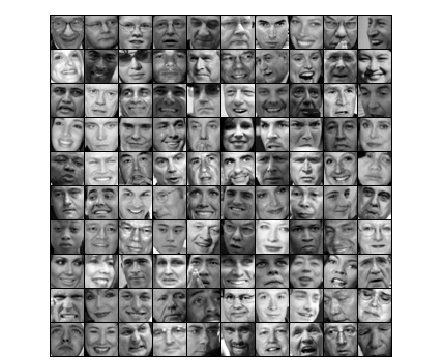1：训练数据处理，归一化。

[X_norm, mu, sigma] = featureNormalize(X);

function [X_norm, mu, sigma] = featureNormalize(X)
%FEATURENORMALIZE Normalizes the features in X
%   FEATURENORMALIZE(X) returns a normalized version of X where
%   the mean value of each feature is 0 and the standard deviation
%   is 1. This is often a good preprocessing step to do when
%   working with learning algorithms.

mu = mean(X);
X_norm = bsxfun(@minus, X, mu);

sigma = std(X_norm);
X_norm = bsxfun(@rdivide, X_norm, sigma);

% ============================================================

end

2：计算协方差矩阵，计算协方差矩阵的特征值

[U, S] = pca(X_norm);

function [U, S] = pca(X)
%PCA Run principal component analysis on the dataset X
%   [U, S, X] = pca(X) computes eigenvectors of the covariance matrix of X
%   Returns the eigenvectors U, the eigenvalues (on diagonal) in S
%

% Useful values
[m, n] = size(X);

% You need to return the following variables correctly.
U = zeros(n);
S = zeros(n);

% ====================== YOUR CODE HERE ======================
% Instructions: You should first compute the covariance matrix. Then, you
%               should use the "svd" function to compute the eigenvectors
%               and eigenvalues of the covariance matrix.
%
% Note: When computing the covariance matrix, remember to divide by m (the
%       number of examples).
%

Sigma = (1/m) *(X'*X);

[U, S, V] = svd(Sigma);

% =========================================================================

end

展示一下前面求的均值mu，一张大众脸。我靠。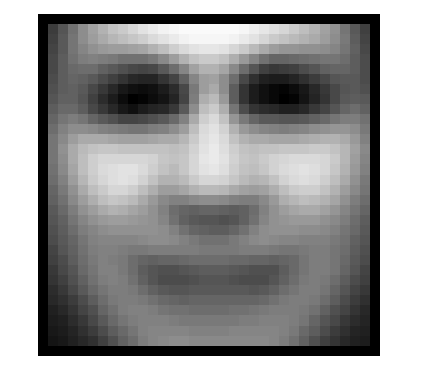展示下特征U，每张都一个脸轮廓。

displayData(U(:, 1:36)');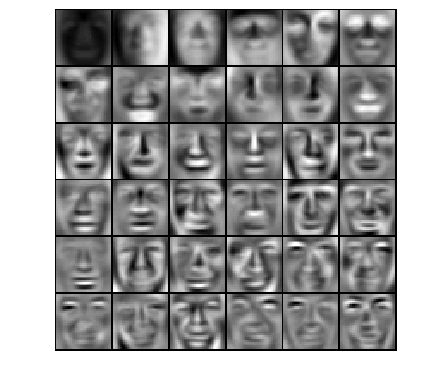3：选取K个维度的特征进行PCA降维。K的选取有公式，这里不阐述。

K = 100;
Z = projectData(X_norm, U, K)

function Z = projectData(X, U, K)
%PROJECTDATA Computes the reduced data representation when projecting only
%on to the top k eigenvectors
%   Z = projectData(X, U, K) computes the projection of
%   the normalized inputs X into the reduced dimensional space spanned by
%   the first K columns of U. It returns the projected examples in Z.
%

% You need to return the following variables correctly.
Z = zeros(size(X, 1), K);

% ====================== YOUR CODE HERE ======================
% Instructions: Compute the projection of the data using only the top K
%               eigenvectors in U (first K columns).
%               For the i-th example X(i,:), the projection on to the k-th
%               eigenvector is given as follows:
%                    x = X(i, :)';
%                    projection_k = x' * U(:, k);
%

Z = X*U(:,1:K);

% =============================================================

end

展示降维的效果，黑黑一片。原来的图片是32*32像素值，现在只有100像素值

displayData(Z(1:100,:));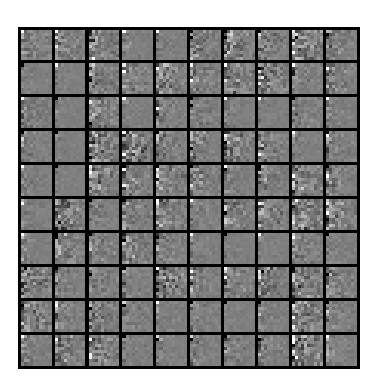4：复原图片

K = 100;
X_rec  = recoverData(Z, U, K);

function X_rec = recoverData(Z, U, K)
%RECOVERDATA Recovers an approximation of the original data when using the
%projected data
%   X_rec = RECOVERDATA(Z, U, K) recovers an approximation the
%   original data that has been reduced to K dimensions. It returns the
%   approximate reconstruction in X_rec.
%

% You need to return the following variables correctly.
X_rec = zeros(size(Z, 1), size(U, 1));

% ====================== YOUR CODE HERE ======================
% Instructions: Compute the approximation of the data by projecting back
%               onto the original space using the top K eigenvectors in U.
%
%               For the i-th example Z(i,:), the (approximate)
%               recovered data for dimension j is given as follows:
%                    v = Z(i, :)';
%                    recovered_j = v' * U(j, 1:K)';
%
%               Notice that U(j, 1:K) is a row vector.
%

X_rec = Z*(U(:,1:K)');

% =============================================================

end

复原后的效果，是不是看上去没什么区别。

% Display normalized data
subplot(1, 2, 1);
displayData(X_norm(1:100,:));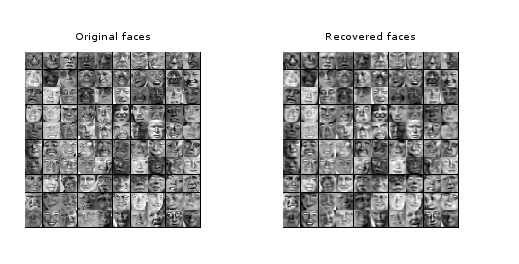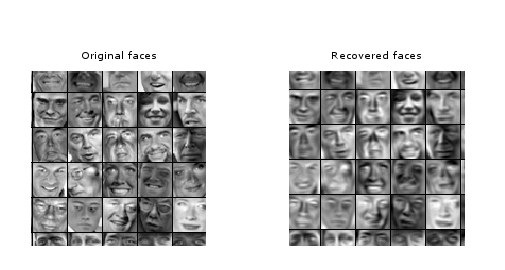一个图片处理的例子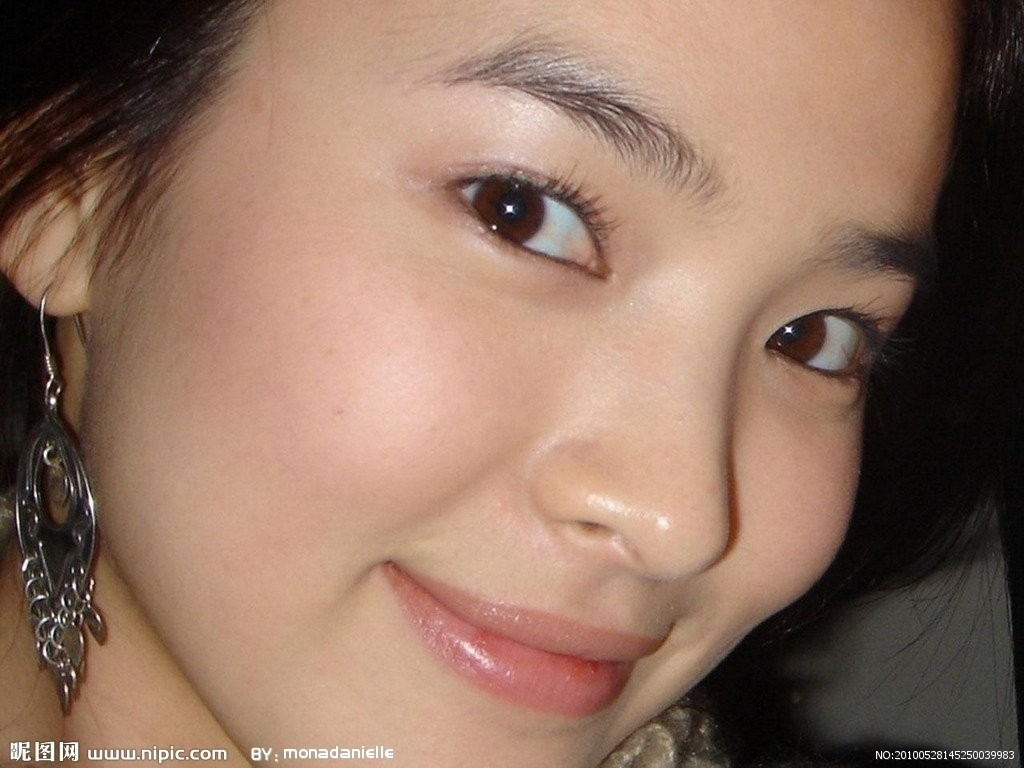python 代码

# -*- coding:utf-8 -*-
from numpy import linalg, mat, dot, eye
from PIL import Image

def main(num=5):
im = Image.open('d:\\s1.jpg')
box = (0, 0, im.size, im.size)
imcopy = im.crop(box)
ma = [[], [], []]
num= int(im.size*1/6)

for i in xrange(3):
for x in xrange(im.size):
ma[i].append([])
for y in xrange(im.size):
ma[i][-1].append(pix[x, y][i])

for i in xrange(3):
u, s, v = linalg.svd(ma[i])
s2 =mat(eye(num)*s[:num])
ma[i]=u[:, :num]*s2*v[:num, :]

for x in xrange(im.size):
for y in xrange(im.size):
ret = []
for i in xrange(3):
tmp = int(ma[i][x,y])
if tmp < 0:
tmp = 0
if tmp > 255:
tmp = 255
ret.append(tmp)
pix[x, y] = tuple(ret)
imcopy.show()

# imcopy.save('d:\\s2.jpg')

if __name__ == '__main__':
main()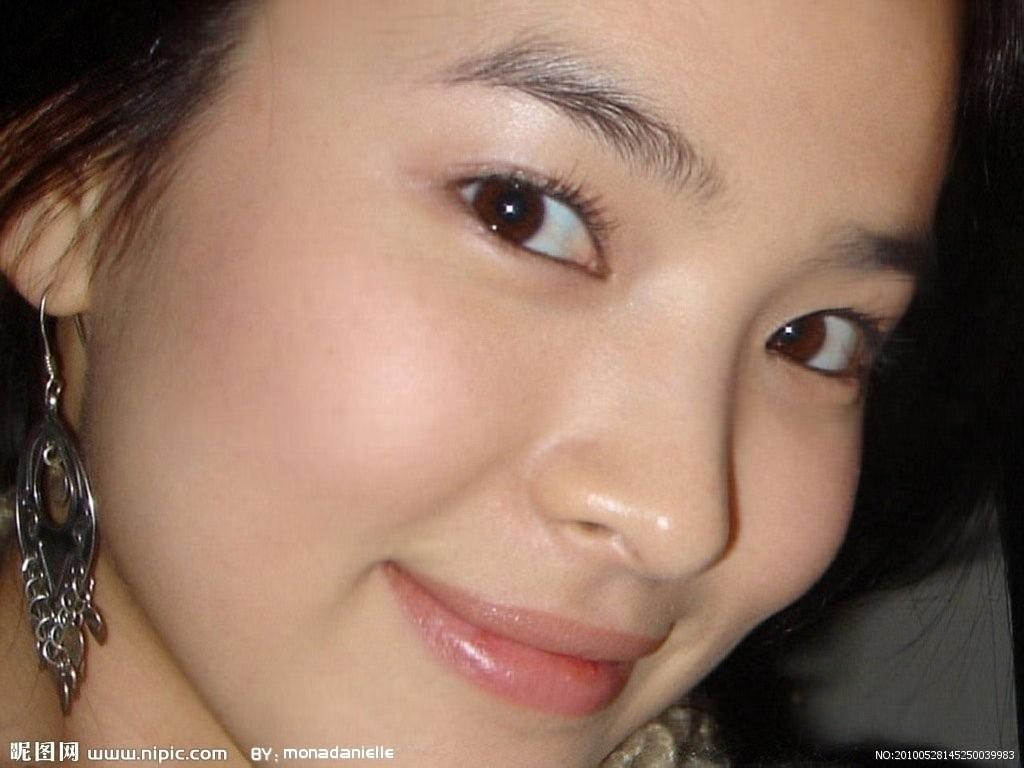http://www.isnowfy.com/introduction-to-svd-and-lsa/

http://blog.csdn.net/redline2005/article/details/24100293

http://blog.csdn.net/moodytong/article/details/10085215

https://share.coursera.org/wiki/index.php/ML:Dimensionality_Reduction

posted on 2016-01-19 17:08  张日海  阅读(3302)  评论(0编辑  收藏  举报